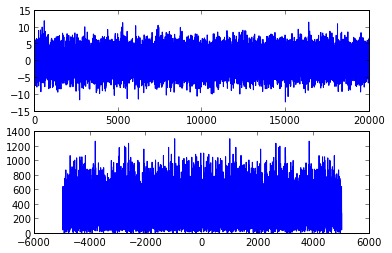# Telecommunications Breakdown Workbook¶

## Chapter 3: The Five Elements¶

### Section 3.1 Finding the Spectrum of a Signal¶

In :
def plotspec(x, Ts):
fig = figure()
ax1.plot(x)

q = fft.fft(x)
ax2.plot(fft.fftfreq(len(x), Ts), abs(q))

In :
# specsquare.py
f = 10.0        # "frequency" of square wave
time = 2.0      # length of time
Ts = 1.0/1000.0 # Sample period
t = linspace(0.0, time, time/Ts) # Create a time vector
x = sign(cos(2*pi*f*t)) # Square wave = sign of cos wave
plotspec(x, Ts)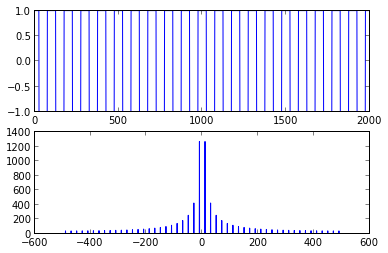In :
def specsquare(f, time, Ts):
t = linspace(0.0, time, time/Ts) # Create a time vector
x = sign(cos(2*pi*f*t)) # Square wave = sign of cos wave
plotspec(x, Ts)


(p42) 3.1 Use specsquare.m to investigate the relationship between the behavior of the square wave and its spectrum.

1. Try square waves with different frequences: f=20, 40, 100, 300 Hz. How do the time pltos change? How do the spectra change?
In :
specsquare(20, 2.0, 1.0/1000.0)
specsquare(40, 2.0, 1.0/1000.0)
specsquare(100, 2.0, 1.0/1000.0)
specsquare(300, 2.0, 1.0/1000.0)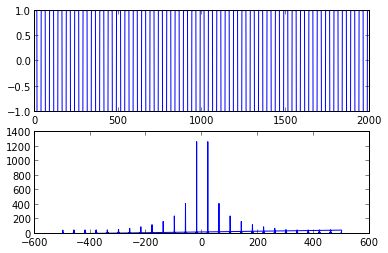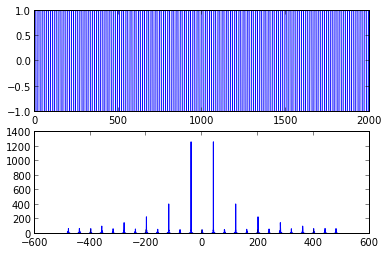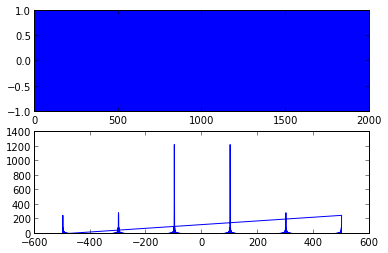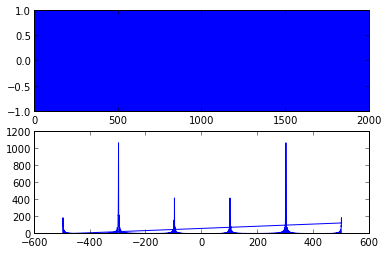How do the spectra change? The tallest peaks move along with the frequency, while the smaller peaks move to occur at the multiples of the base frequency. At 300Hz, weird peaks pop up at 100Hz.

Some artifacting in the graph itself, probably due to an odd/even problem with the FFT or some such (?)

1. Try square waves of different lengths, time=1,10,100s. How does the spectrum change in each case?
In :
specsquare(20, 1.0, 1.0/1000.0)
specsquare(20, 2.0, 1.0/1000.0)
specsquare(20, 10.0, 1.0/1000.0)
specsquare(20, 100.0, 1.0/1000.0)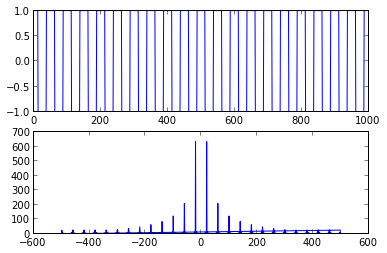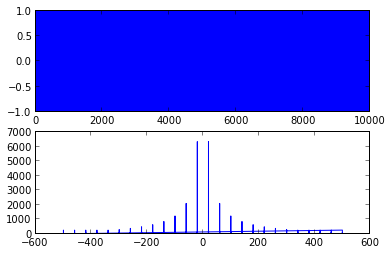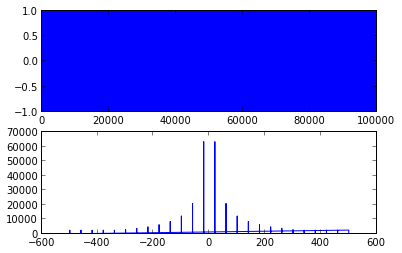No apparent change in spectra due to lengthening the window.

1. Try different sampling rates: Ts=1/100, 1/10000 s. How do the spectra change?
In :
specsquare(20, 2.0, 1.0/100.0)
specsquare(20, 2.0, 1.0/1000.0)
specsquare(20, 2.0, 1.0/10000.0)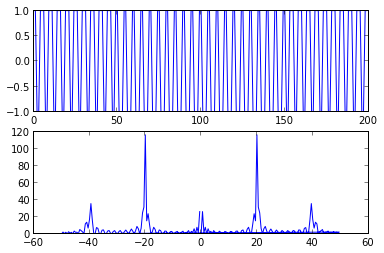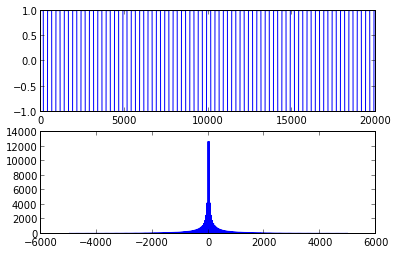At low sample rates, we see lots of noise due to Nyquist folding of the higher-frequency components of the square wave. At the higher sample rate, we get a very pretty curve showing the drop-off in the power of the higher frequency components.

3.3 Mimic specsquare.py to find spectra of:

In :
def arbspec(s, time, Ts):
t = linspace(0.0, time, time/Ts)
x = s(t)
plotspec(x, Ts)

In :
def exppulse(t):
return exp(-1*t)
arbspec(exppulse, 20.0, 1.0/100.0)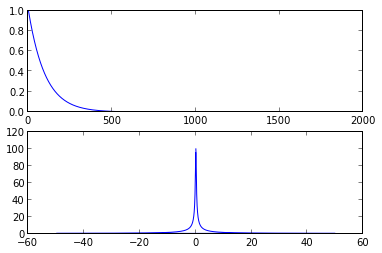In :
def gaussianpulse(t):
return exp(-1*(t**2))
arbspec(gaussianpulse, 20.0, 1.0/100.0)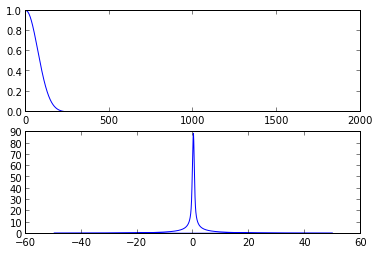In :
def sinusoidgen(f, phi):
def s(t):
return sin(2*pi*f*t+phi)
return s

for f in [20,100,1000]:
for phi in 0, pi/4.0, pi/2.0:
arbspec(sinusoidgen(f,phi), 2.0, 1.0/1000.0)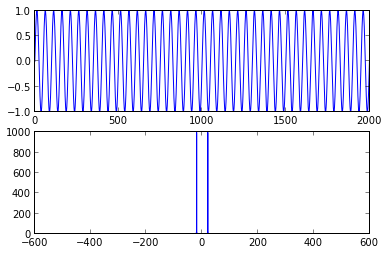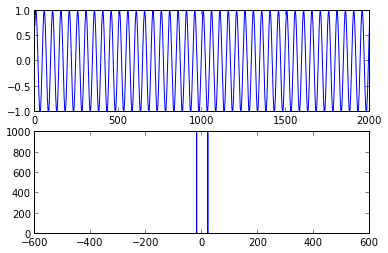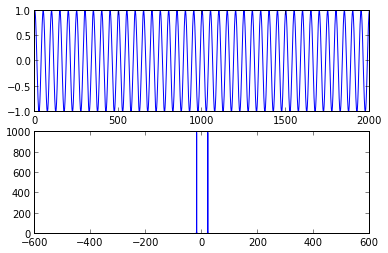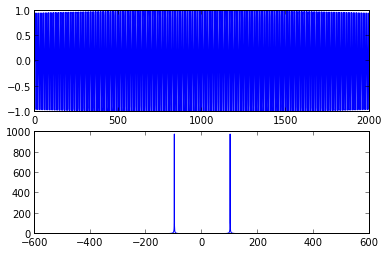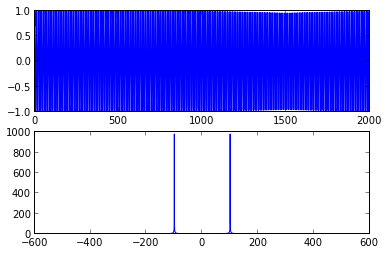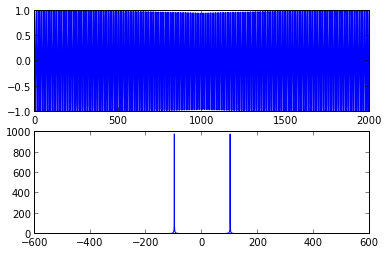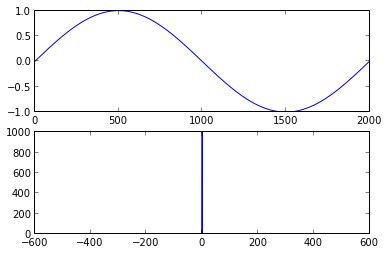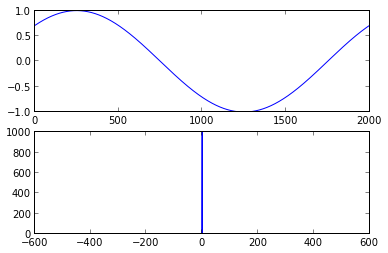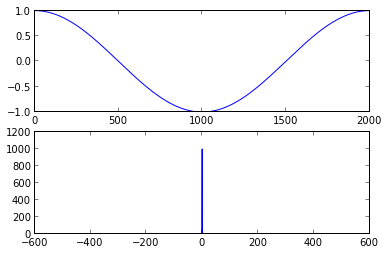3.4 MATLAB has several commands that create random numbers:

1. Use rand to create a signal that is uniformly distributed on [-1,1]. Find the spectrum of the signal.
In :
def noise(t):
return random.uniform(-1,1,len(t))
arbspec(noise, 2.0, 1.0/10000.0)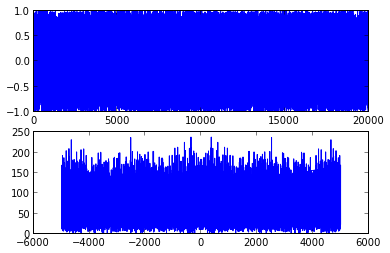1. use rand and sign to make a signal that is +1 with P=1/2 and -1 with P=1/2. Find the spectrum
In :
def signnoise(t):
return sign(random.uniform(-1,1,len(t)))
arbspec(signnoise, 2.0, 1.0/10000.0)

# Double-check that we're maintaining the P=0.5 condition
figure(); hist(signnoise(linspace(0,2,20000)))

Out:
(array([ 9919,     0,     0,     0,     0,     0,     0,     0,     0, 10081]),
array([-1. , -0.8, -0.6, -0.4, -0.2,  0. ,  0.2,  0.4,  0.6,  0.8,  1. ]),
<a list of 10 Patch objects>)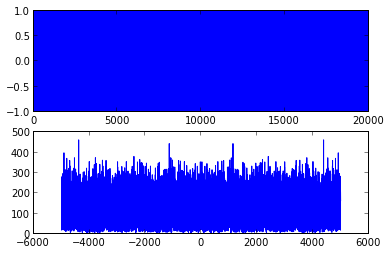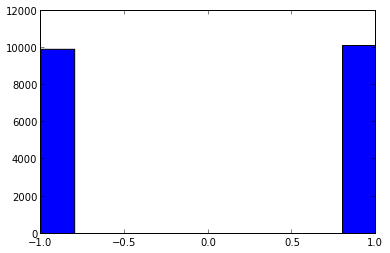1. Use randn to create a signal that is normally distributed with mean=0 and variance 3. Find the spectrum of the signal
In :
def gaussiannoise(t):
return random.normal(0.0, 3.0, len(t))

arbspec(gaussiannoise, 2.0, 1.0/10000.0)